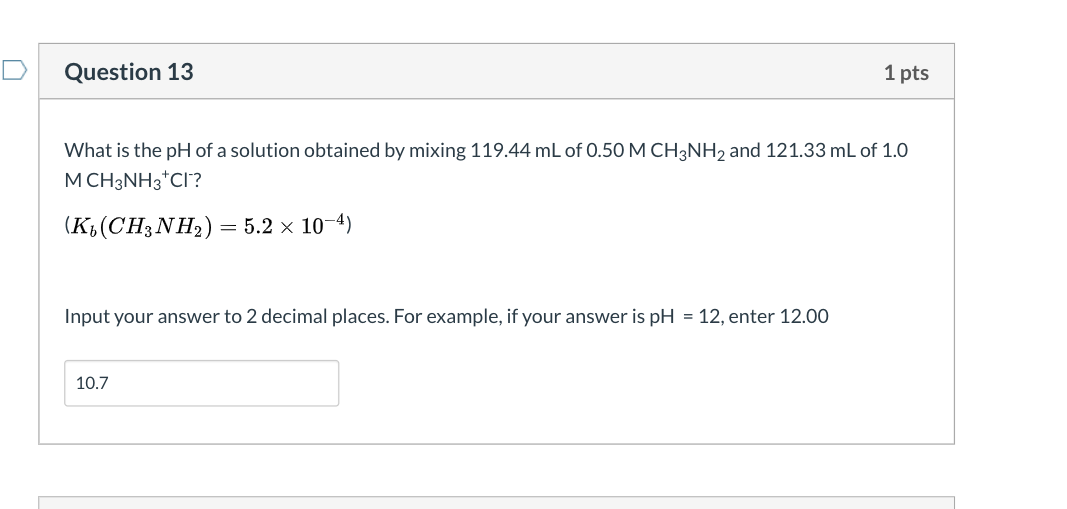# Question Solved1 AnswerQuestion 13 1 pts What is the pH of a solution obtained by mixing 119.44 mL of 0.50 M CH3NH2 and 121.33 mL of 1.0 MCH3NH3CI? (K (CH3NH2) = 5.2 x 10-4) Input your answer to 2 decimal places. For example, if your answer is pH = 12, enter 12.00 10.7Transcribed Image Text: Question 13 1 pts What is the pH of a solution obtained by mixing 119.44 mL of 0.50 M CH3NH2 and 121.33 mL of 1.0 MCH3NH3CI? (K (CH3NH2) = 5.2 x 10-4) Input your answer to 2 decimal places. For example, if your answer is pH = 12, enter 12.00 10.7
More
Transcribed Image Text: Question 13 1 pts What is the pH of a solution obtained by mixing 119.44 mL of 0.50 M CH3NH2 and 121.33 mL of 1.0 MCH3NH3CI? (K (CH3NH2) = 5.2 x 10-4) Input your answer to 2 decimal places. For example, if your answer is pH = 12, enter 12.00 10.7